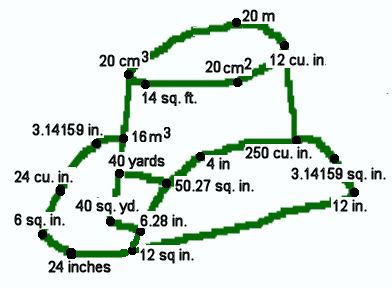Name ___________________________________________Connect the answers in order.   1. 12 sq in. The area of a rectangle with a base of 4 in. and a height of 3 in. 2. 12 in. The perimeter of a 2 in. by 4 in. rectangle. Start a new line. 3. 12 cu. in. The volume of a square pyramid, 4 in. by 4 in. by 3 in. 4. 250 cu. in. The volume of a hexagonal pyramid having a base area of 75 sq. in. and a height of 10 in. Start a new line. 5. 20 cm3 The volume of a prism that is 2cm by 2cm by 5 cm. 6. 14 sq. ft. The area of a trapezoid with bases 3 and 11 ft. and a height of 2 ft. 7. 20 cm² The area of a parallelogram that is 4 cm in height and has a base of 5 cm. 8. 12 cu. in. The volume of a pyramid with a 2 in by 3 in base that is 6 in tall. 9. 20 m The perimeter of a square 5 m on a side. 10. 20 cm3 The volume of a rectangular pyramid with length, width, and height of 3 cm, 5 cm, and 4 cm. 11. 40 yards The perimeter of a rectangle 2 1/2 yards by 8 yards. 12. 40 sq. yd. The area of a triangle with a base of 8 yd. and a height of 10 yd. 13. 6.28 in. The perimeter of a circle radius 1 inch. Start a new line. 14. 12 sq in. The area of a triangle with a base of 8 in., and a height of 3 in. 15. 24 inches The perimeter of a square whose area is 36 sq. in. 16. 6 sq. in. The area of 3 in. by 4 in. by 5 in. triangle. 17. 24 cu. in. The volume of a pyramid with base sides of 4 in. and 3 in., and a height of 6 in. 18. 3.14159 in. The circumference of a circle with a 1 inch radius. 19. 16 m3 The volume of a square pyramid with a height of 3m and a 4 m by 4 m base. Start a new line. 20. 12 in. The base of rectangle with an area of 48 sq. in. and height of 4 in. 21. 3.14159 sq. in. The area of a circle with a radius of 1 in. 22. 250 cu. in. The volume of a 5 x 5 x10 in. prism. 23. 4 in The side of a square whose area is 16 sq. in. 24. 50.27 sq. in. The area of a circle with diameter of 8 in. 25. 6.28 in. The circumference of a circle with diameter of 1 in. 26. 12 sq in. The area of a triangle with a height of 8 in. and a base of 3 in. Start a new line. 27. 40 yards The height of triangle with an area of 240 sq. yd and a base of 12 yd. 28. 50.27 sq. in. The area of a circle with a radius of 4 in.